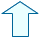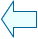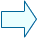CONSTRUCTING TRIANGLES Geometry

1. GIVEN THE THREE SIDES.

If we know the length of its three sides we can draw a triangle. However, knowing the length of the sides alone is not enough to be able to draw some non-regular quadrilaterals or non-regular polygons.  In the following window we are going to see how a triangle can be constructed when the three sides are given. However, this is not possible when one of the sides is greater than the sum of the other two.

1.- Use a ruler and compass to draw a triangles whose sides are 6 cm, 9 cm and 4 cm long. Draw a triangle whose three sides are 7cm long. Try drawing a triangle whose sides are 7 cm, 3 cm and 12 cm long.

2.-Construct the triangles in this Descartes window.

 Use the buttons in the lower part of the window to change the lengths of the sides, click on clear and then move the two red end points with the mouse until you draw two arcs that intersect at a point. The triangle is defined when these two points join together.

2. GIVEN THREE ANGLES.

You will soon find there are an infinite amount of triangles that can be constructed with the  three angles that are given (knowing two angles is enough as we know that the three add up to 180º).

 Click on the Init button in this window and write down the values given for the three sides in your exercise book. Then, drag point C with the mouse until side a is equal to 10 and 16 and write down the lengths of the sides in each case.

3.- Click on the Init button and repeat the process for B=115º and C=25º.

4.- Also, draw three right-angled triangles, which are isosceles triangles, and three equilateral triangles and write down the length of the sides of each one in your exercise book.

3. Given two sides and the included angle.
You can can also construct a triangle if you know the length of two of the sides and the included angle (the angle the two sides share). In this case constructing the triangle is very straightforward as the third side is automatically made by drawing a line to join the ends of the other two sides together.

5.- Use this window to construct the following triangle in your exercise book: a=5, b=6 and angle C=47º

 Introduce the three values in the correct space and the window will work out the rest.

6.- Repeat the process for a triangle with sides a=8, b=5 and angle C=132º

7.-  In the same way draw an equilateral triangle whose sides are 6 units long and a right-angled triangle whose shortest sides are 3 and 4 units long.

 4.Given one side and two adjacent angles. Finally, if we know the length of one side and the size of two adjacent angles only one possible triangle can be constructed. In the following window you can see the one triangle that can be constructed for different values of a, B and C. 6.- Use this window to help you construct the following triangles in your exercise book: a=7, C=45º, B=50º; a=9, C=25º, B=120º; and an equilateral triangle whose sides are 10 units long and three isosceles triangles which have one side which is 8 units long.Miguel García ReyesSpanish Ministry of Education. Year 2001